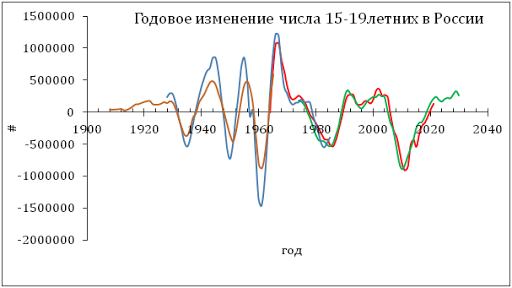## 6/15/11

### Oil price will be decreasing through the rest of 2011. CPI vs core CPI

The U.S. Bureau of Labor Statistics has reported the estimates of various consumer price indices for May 2011. According to our schedule, we have to revisit the difference between the headline and core CPI only in July 2011. However, the new estimates likely manifest a short-term turn in the difference which is worth mentioning.
Figures 1 and 2 briefly introduce our concept of sustainable (quasi-linear) long-term trends in the difference between the headline and core CPI in the U.S. There were two clear periods of linear behaviour: between 1981 and 1999 and between 2002 and 2009. A natural assumption of the future evolution of the difference was that a new trend has to emerge around 2010 after a short period of very high volatility.
Figure 1. Linear regression of the difference between the core CPI and CPI for the period from 1981 to 1999 (R2= 0.96 the slope is 0.67) and linear regression of the difference between the core CPI and CPI between 2002 and 2009 (R2=0.91, and the slope is -1.59).
Accordingly, Figure 2 illustrate this hypothesis with the reversion (like mirror reflection) of the trend between 2002 and 2009. We expected this new trend with a positive slope to be developed between 2008 and 2011, as shown by the solid red line. Against our expectations, after a year of “right” evolution in 2010 the difference fell to the zero line again.
Figure 2. The evolution of the difference between the core and headline CPI since 2002.
The May 2011 estimates suggest the end of the fall in the difference and a pivot to the long-term trend (solid red line in Figure 2). Figure 3 depicts the most recent period with a clear turn in May 2011. Thus, we expect the difference will return to the long-term trend by the end of 2011. This return should be accompanied by a remarkable drop in the index price of energy which was the driver of the headline CPI in 2011.  Hence, ol price will be falling during the rest of 2011.

Figure 3. The evolution of the difference between the core and headline CPI since 2010.